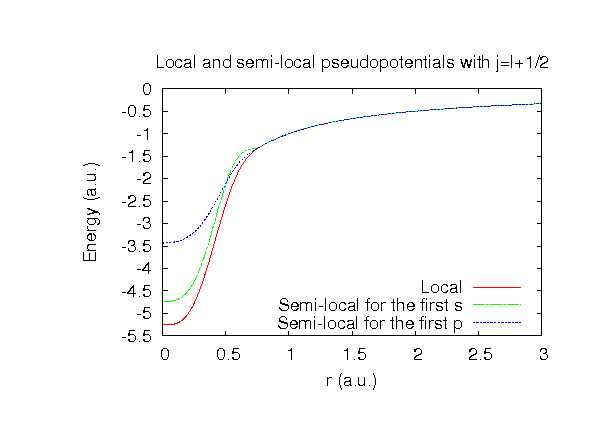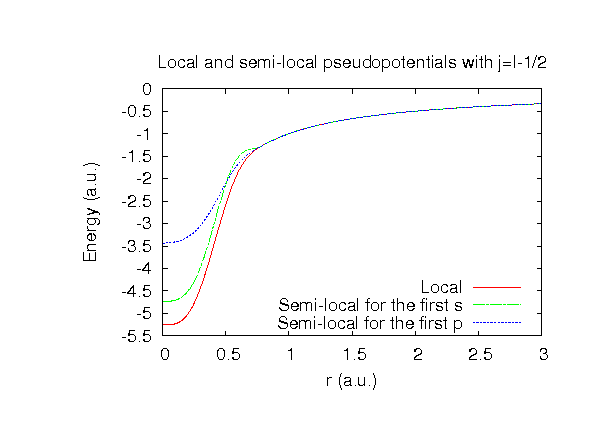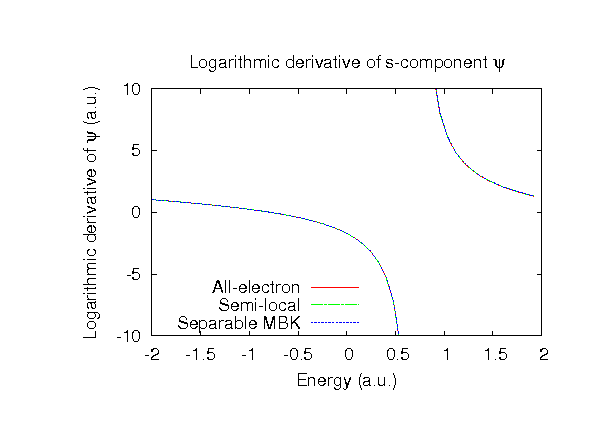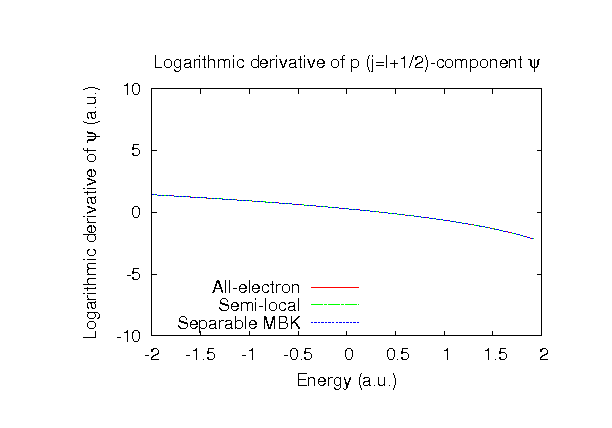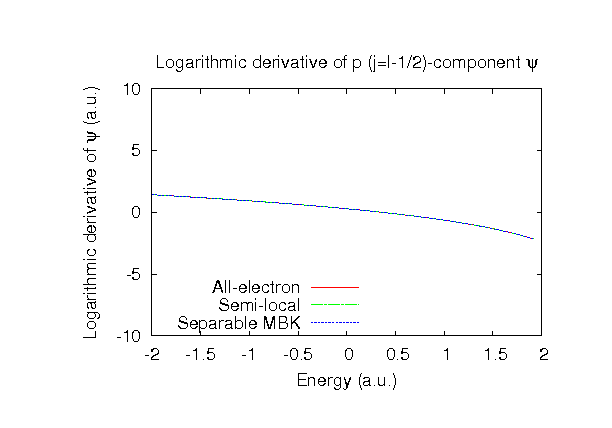### Fully relativistic pseudopotentials

Fully relativistic pseudopotentials generated by the MBK (PRB 47, 6728 (1993)) scheme within LDA (CA13) and GGA (PBE13) which contain a partial core correction and fully relativistic effects including spin-orbit coupling, while the partial core correction is zero for a hydrogen atom.

### Pseudo-atomic orbitals

The number below the symbol means a cutoff radius (a.u.) of the confinement potential. These file includes fifteen radial parts for each angular momentum quantum number l (=0,1,2,3). The basis functions were generated by variationally optimizing in three environments: the single atom, a water molecule, and the dimer molecule. The input files used for the orbital optimization can be found at H_opt.dat , H2O_opt.dat, and H2_opt.dat . Since H_CA13.vps and H_PBE13.vps include the 1s state (1 electron) as the valence state, the minimal basis set is H*.*-s1. Our recommendation for the choice of cutoff radius of basis functions is that H5.0.pao is enough for bulks, but H6.0.pao or H7.0.pao is preferable for molecular systems.

### Benchmark calculations by the PBE13 pseudopotential with the various basis functions

(1) Calculation of the total energy as a function of lattice constant in the diamond structure, where the total energy is plotted relative to the minimum energy for each case. a0 and B0 are the equilibrium lattice constant and bulk modulus obtained by fitting to the Murnaghan equation of state. The difference between H5.0-s2p1 and H5.0-s3p2 in the total energy at the minimum point is 0.012 eV/atom. An input file used for the OpenMX calculations can be found at Hdia-EvsV.dat . For comparison the result by the Wien2k code is also shown, where the calculation was performed by default setting in the Ver. 10.1 of Wien2k.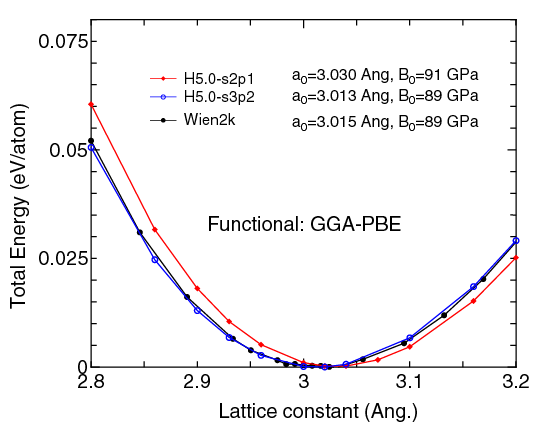(2) Calculations of the band dispersion in the diamond structure, where the non-spin polarized collinear calculation with the lattice constant of 3.0 Ang. was performed using H_PBE13.vps and H5.0-s2p1 or H5.0-s3p2 and the origin of the energy is taken to be the Fermi level. The input file used for the OpenMX calculations can be found at Hdia-Band.dat . For comparison the result by the Wien2k code is also shown, where the calculation was performed by default setting in the Ver. 10.1 of Wien2k.(3) Calculations of a hydrogen molecule, where H_PBE13.vps and H7.0-s2p1 or H7.0-s3p2 were used. The input files used for the OpenMX calculations can be found at H2.dat , H.dat , and H_cp.dat .

 Equilibrium bond length (Ang.) Atomization energy (eV) Atomization energy (couterpoise corrected) (eV) H7.0-s2p1 0.752 4.51 4.51 H7.0-s3p2 0.750 4.56 4.56 Other calc. 0.750a 4.54b Expt. 0.741c 4.75c

a T. Mueller and G. Ceder J. Phys. Chem. B 109, 17974 (2005).
b S. Kurth et al., Int. J. of Quantum Chem. 75, 889 (1999).
c K.P. Huber and G. Herzberg, Constants of Diatomic Molecules (Van Nostrand Reinhold, New York, 1979).

(4) Calculations of a water molecule, where O_PBE13.vps, H_PBE13, O7.0-s2p2d1, O7.0-s3p3d2, H7.0-s2p1, and H7.0-s3p2 were used. The input files used for the OpenMX calculations can be found at H2O.dat .

 Equilibrium H-O bond length (Ang.) Equilibrium H-O-H bond angle (Deg.) Dipole moment (Debye) O7.0-s2p2d1, H7.0-s2p1 0.971 103.7 1.82 O7.0-s3p3d2, H7.0-s3p2 0.970 103.8 1.83 Other calc. 0.969a 104.2a 1.81b Expt. 0.957c 104.5d 1.86 c

a A.E. Mattsson and T.R. Mattsson, J. Chem. Theory Comput. 5, 887 (2009).
b X. Xu and W.A. Goddard III, J. Phys. Chem. A 108, 2305 (2004).
c W. S. Benedict, N. Gailar, and E. K. Plyler, J. Chem. Phys. 24, 1139 (1956).
d S. A. Clough, Y. Beers, G. P. Klein, and L. S. Rothman, J. Chem. Phys. 59, 2254 (1973).

(5) Calculations of a water dimer, where O_PBE13.vps, H_PBE13, O7.0-s2p2d1, O7.0-s3p3d2, H7.0-s2p1, and H7.0-s3p2 were used. The input files used for the OpenMX calculations can be found at H2O.dat , d-H2O.dat , H2O_cp1.dat , and H2O_cp2.dat ,

 Equilibrium O-O distance (Ang.) Dipole moment (Debye) Binding energy (kcal/mol) Binding energy (couterpoise corrected) (kcal/mol) O7.0-s2p2d1, H7.0-s2p1 2.899 2.54 5.57 5.21 O7.0-s3p3d2, H7.0-s3p2 2.897 2.45 5.48 5.48 Other calc. 2.893a 5.15 a Expt. 2.98b 2.60b 5.44 b

a A.E. Mattsson and T.R. Mattsson, J. Chem. Theory Comput. 5, 887 (2009).
b F. Sim, A. St.-Amant, I. Papai, and D. R. Salahub, J. Am. Chem. Soc. 114, 4391 (1992).

### Supplementary information for the GGA (PBE13) pseudopotential